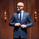3561 views
3561
Source code that allows to find bullish or bearish pin bars in any asset.
```//@version=2
study("Pin bar")

xor (a, b) =>
(a and not b) or (not a and b)

long_bar(complete_body, tail_length) =>
(tail_length > 3 * complete_body)

bullish_bar(third_part_pos) =>
complete_body_length = 0
tail_length = 0

is_pin_bar = (open >= third_part_pos) and (close >= third_part_pos)

bullish_body = close > open

if open > close
complete_body_length := high - close
tail_length := close - low
else
complete_body_length := high - open
tail_length := open - low

long_tail = long_bar(complete_body_length, tail_length)? true: close < open

is_pin_bar and (long_tail or bullish_body)

bearish_bar(third_part_pos) =>
complete_body_length = 0
tail_length = 0

is_pin_bar = (open <= third_part_pos) and (close <= third_part_pos)

bearish_body = close < open

if open > close
complete_body_length = high - close
tail_length = close - low
else
complete_body_length = high - open
tail_length = open - low

long_tail = long_bar(complete_body_length, tail_length)? true: close < open

is_pin_bar and (long_tail or bearish_body)

isPinBar() =>
range = high - low
third_part_range = range / 3
third_part_pos_bullish = high - third_part_range
third_part_pos_bearish = low + third_part_range

bullish_pin_bar = bullish_bar(third_part_pos_bullish)
bearish_pin_bar = bearish_bar(third_part_pos_bearish)

result = xor(bullish_pin_bar, bearish_pin_bar)? 1: 0

if result and bearish_pin_bar
result := -1

result

barcolor(isPinBar() == 1 or isPinBar() == -1? red: na)
plot(isPinBar())```You should display something on the chart rather than ading that extra indicator space on the bottom, it take a lot of space that could be used for an other indicator.